## Numerical - North West Corner Method

The North West corner method is one of the methods to obtain a basic feasible solution of the transportation problems (special case of LPP).

We will now see how to apply this very simple method to a transportation problem. We will study steps of this method while applying it in the problem itself.

### Numerical

#### A mobile phone manufacturing company has three branches located in three different regions, say Jaipur, Udaipur and Mumbai. The company has to transport mobile phones to three destinations, say Kanpur, Pune and Delhi. The availability from Jaipur, Udaipur and Mumbai is 40, 60 and 70 units respectively. The demand at Kanpur, Pune and Delhi are 70, 40 and 60 respectively. The transportation cost is shown in the matrix below (in Rs). Use the North-West corner method to find a basic feasible solution (BFS).

Note that all the explanation is provided in “CYAN” colour. You have to write in examination the only thing which are given in this regular colour under each steps(if any), else you can directly solve matrix of the problem as explained here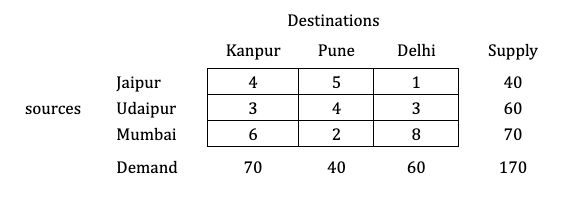Solution:

#### Step 1: Balance the problem

Balance the problem meaning we need to check that if;

$\color{#32c5d4} \Sigma \text { Supply} = \Sigma \text { Demand}$

If this holds true, then we will consider the given problem as a balanced problem.
Now, what if it’s not balanced?

$\color{#32c5d4} \text {i.e., } \Sigma \text { Supply} \not = \Sigma \text { Demand}$

If such a condition occurs, then we have to add a dummy source or market; whichever makes the problem balanced. You can watch a video on this type of numerical, which is known as Unbalanced Transportation Problems.

$\to$ The given transportation problem is balanced.

#### Step 2: Start allocating from North-West corner cell

We will start the allocation from the left hand top most corner (north-west) cell in the matrix and make allocation based on availability and demand.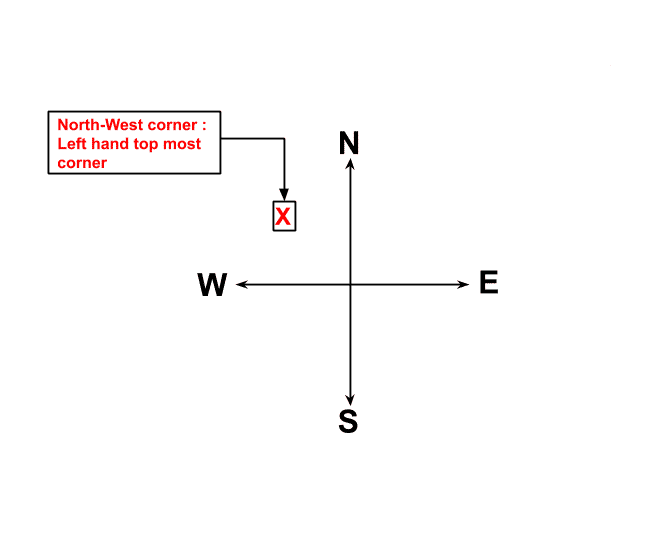Now, verify the smallest among the availability (Supply) and requirement (Demand), corresponding to this cell. The smallest value will be allocated to this cell and check out the difference in supply and demand, representing that supply and demand are fulfilled, as shown below.#### Step 3: Remove the row or column whose supply or demand is fulfilled and prepare a new matrix

As we have fulfilled the availability or requirement for that row or column respectively, remove that row or column and prepare a new matrix, as shown below.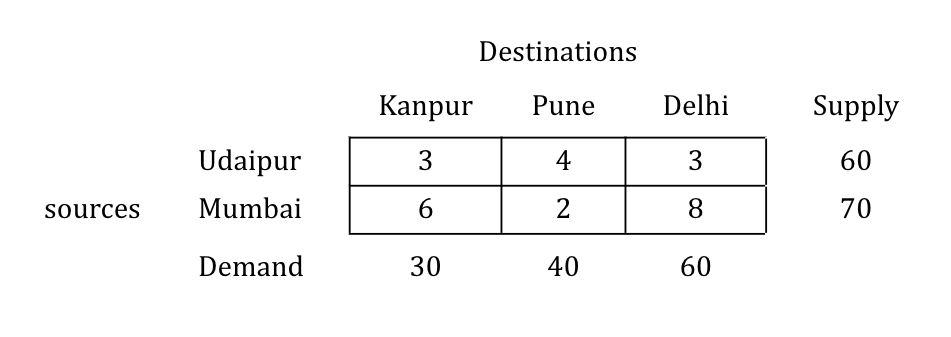#### Step 4: Repeat the procedure until all the allocations are over

Repeat the same procedure of allocation of the new North-west corner so generated and check based on the smallest value as shown below, until all allocations are over.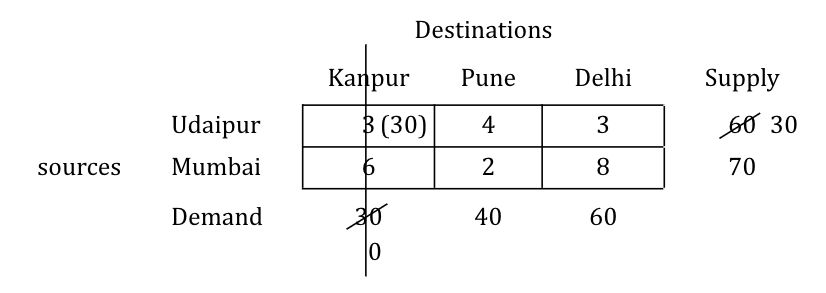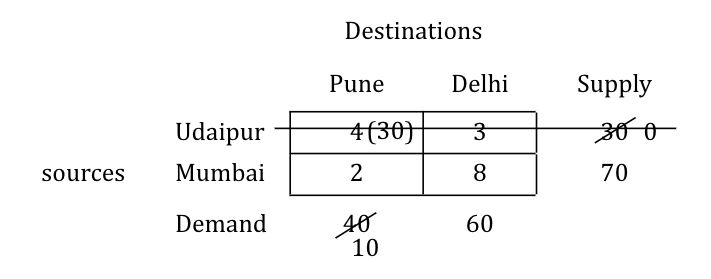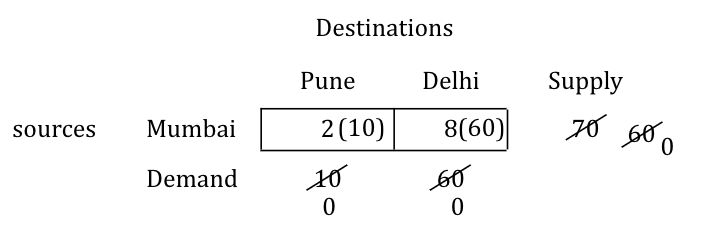#### Step 5: After all the allocations are over, write the allocations and calculate the transportation cost

Once all allocations are over, prepare the table with all allocations marked and calculate the transportation cost as follows.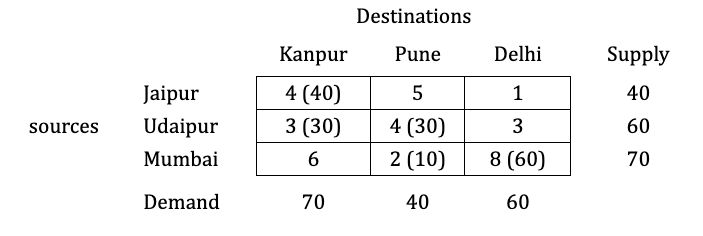\begin{aligned} \to \ \text {Transportation cost} &= (40 \times 40) + (3 \times 30) + (4 \times 30) + (2 \times 10) + (8 \times 60) \\ &= \text {Rs } 870 \end{aligned}

Find solution of same numerical by: